Science Research
Volume 4, Issue 2, April 2016, Pages: 67-71

Numerical Simulations of Current and Wave Around a Circular Cylinder

Congfang Ai*, Weiye Ding, Sheng Jin

State Key Laboratory of Coastal and Offshore Engineering, Dalian University of Technology, Dalian, China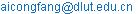(Congfang Ai)(Weiye Ding)

*Corresponding author

Congfang Ai, Weiye Ding, Sheng Jin. Numerical Simulations of Current and Wave Around a Circular Cylinder. Science Research. Vol. 4, No. 2, 2016, pp. 67-71. doi: 10.11648/j.sr.20160402.18

Received: March 23, 2016; Accepted: April 28, 2016; Published: May 24, 2016

Abstract: This paper presents numerical simulations of a circular cylinder under the action of current or wave only. The model solves the three-dimensional (3D) Reynolds-averaged Navier-Stokes equations using an explicit projection method. The 3D grid system is made of lots of prisms, which are built from a two-dimensional horizontal triangular grid by adding a number of horizontal layers. A non-linear k-ε model, which can take into account the anisotropy of turbulence is incorporated as a turbulence model. Two test cases including the current flows around and regular waves interact with a circular cylinder are used to demonstrate the capability of the model. The model gives reasonable results in comparison with available experimental data.

Keywords: Circular Cylinder, Turbulence Model, Numerical Simulation, Three-Dimensional Model

Contents

1. Introduction

The study of current or wave interacting with a circular cylinder has been of interest for many decades. It is well-known that flows around the cylinder induced by the current or wave are very complicated. For example, horseshoe vortex in front of the cylinder and vortex shedding in the back region of the cylinder may be generated. The presence of the cylinder also can induce the contraction of streamlines, and as a result the amplification of the bed shear stress is produced, which has very important effect on the scour around the cylinder. In a word, the knowledge of the flow generated around cylinders is very essential in the design of coastal and oceanic vertical structures.

To date, many numerical predictions have been performed to study the interaction between a current and a cylinder. For example, Onget al. (2009) investigated high Reynolds number flows around a smooth circular cylinder  using 2D unsteady Reynolds-averaged Navier-Stokes equations with a standard high Reynolds number k-ε turbulence model. Salih Kirkgoz et al. (2009) presented numerical simulations of 2D turbulent flow around a smooth horizontal circular cylinder near a rigid bed with gap ratio G/D=0.3 . The Ansys 10.0-FLOTRAN program package is used to solve the governing equations by FEM, and the performance of different turbulence models are examined. For the study of the interaction between wave and a cylinder, there are also many numerical models. Boussinesq equation models are depth-averaged two-dimensional (2D) models and have been extensively used for the study of wave-induced run-up and forces on cylinders (Li et al., 1999; Li and Zhan, 2001 ; Zhao et al., 2007 ; Zhong and Wang, 2009 ). In addition, potential flow models are the other class of widely-used numerical models. For example, Ma et al. (2001a, 2001b) developed a fully nonlinear finite element model for simulating the interactions between fixed vertical cylinders and steep waves [8, 9]. Wang and Wu (2010) studied the nonlinear interactions between water waves and vertical cylinder arrays in a finite-element based numerical tank . However, although considerable numerical models have been applied to study current or wave interacting with a cylinder, it is very rare that a numerical model has the capability of simultaneously predicting a current interacting with a cylinder and the interaction between wave and a cylinder.

In this paper, we proposed a 3D numerical model to predict flows around a circular cylinder under the action of current or wave only. The model is developed based upon a former model (Ai and Jin, 2010 ), which has been successfully applied to study solitary waves interacting with structures. A non-linear k-ε model is adopted in the model as a turbulence model.

2. Governing Equations

The governing equations are the 3D Reynolds-averaged Navier-Stokes (RANS) equations, which are based on the conservation of mass and momentum and can be expressed as: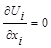(1)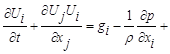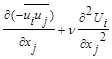(2)

where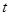is the time;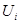are the time-averaged velocity components;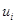are the turbulent velocity components;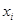is the spatial coordinate;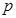is the normalized pressure divided by a constant reference density; and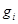are the gravitational accelerations.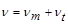(3)

where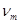is the molecular kinematic viscosity;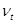is the eddy viscosity and is determined by the following non-linear-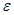turbulence model (Kimura and Hosoda, 2003 )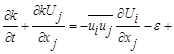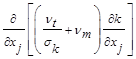(4)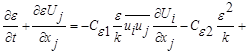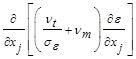(5)

whereis the averaged turbulent energy andis the averaged turbulent energy dissipation rate. The Reynolds stress tensors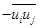in Eqs. (2), (4) and (5) are evaluated by the following non-linear expression instead of the linear equation used in the standard-model.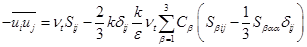(6)

where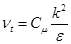(7)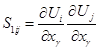(8)(9)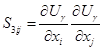(10)

The coefficients in Eqs. (6) and (7) are expressed as follows: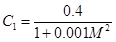(11)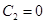(12)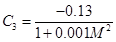(13)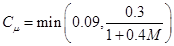(14)

in which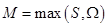(15)

and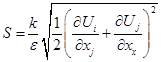(16)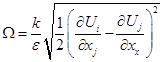(17)

3. Boundary Conditions

Boundary conditions are required at all the boundaries of a three-dimensional domain including the free surface and the bottom. At the moving free surface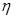, the kinematic boundary condition is(18)

In addition, atmospheric pressure is assumed at free surface elevation, giving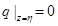(19)

For the impermeable bottom surface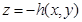, the kinematic bottom boundary condition is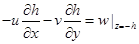(20)

Using kinematic boundary conditions (18) and (20) in the integrated form of the continuity equation (1) over the water column, the free surface equation is obtained: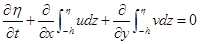(21)

At rigid wall boundaries, velocity normal to the wall is zero and the tangential velocity is determined by the wall-function approach. In this study, only smooth rigid walls are considered, so velocity parallel to the wall is described by the following logarithmic law: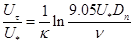(22)

where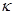is von Karman’s constant;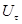is velocity parallel to the smooth wall;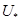is the shear velocity; and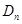is the normal distance from the wall.

At the free surface, the boundary conditions for turbulent quantities are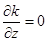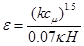(23)

where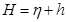is the water depth.

At the rigid walls, the following boundary conditions for turbulent quantities are specified.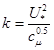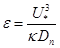(24)

At inflow boundaries, the velocity normal to the boundary and turbulent quantities are specified as follows: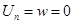(25)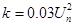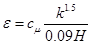(26)

4. Numerical Methods

The overall numerical algorithm consists of the following three steps.

The first step is to solve the RANS by using an explicit projection method, which is subdivided into two stages (Ai et al., 2011 ).

The first stage is to project intermediate velocities by means of solving the momentum equations that contain the non-hydrostatic pressure at the previous time level. In this stage, the governing equations (1) and (2) are firstly integrated over a vertical layer. Then, the resulting momentum equations in question are obtained by subtracting the integrated continuity equation from the integrated momentum equations. Finally, a finite volume method with a first-order upwind scheme is used to discretize the advection terms of the momentum equations. The resulting discretized equations guarantee conservation of momentum.

In the second stage, the new velocities are computed by correcting the projected values after including the non-hydrostatic pressure terms, which are obtained by solving the discretized Poisson equation. It is worth mentioning that, because of a new grid arrangement employed in the present model, the Poisson equation is symmetric and positive definite and thus can be solved efficiently by preconditioned conjugated gradient method. In this study, the symmetric Gauss-Seidel method is used as the preconditioner to solve the Poisson equation.

In the second step, by substituting the resulting velocities into a discretized form of the free surface equation (21), we can obtain the new free surface elevation.

Finally, we employed finite volume method to discretize the Eqs. (4) and (5) to get the turbulence quantities.

More details about the numerical solution can be found in the references (Ai and Jin, 2010 ; Ai et al., 2011 ; Ai and Jin, 2012  ;Ai et al., 2014 ).

5. NumericalResults

5.1. The Flow Around a Vertical Circular Cylinder Exposed to a Steady Current

In order to examine the capability of the model to simulate a current interacting with a circular cylinder, the model validation was achieved against the experimental data obtained by Roulund et al. (2005)  in the first test case, which has been validated by Zhang Bojie et al. (2015) . The experiment was conducted in a flume with 35 m long and 3 m wide. The water depth was maintained at 0.54 m. The cylinder diameter was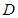= 0.536 m. A schematic diagram is plotted in Fig. 1. In the simulation, a constant inflow velocity of 0.326 m/s was imposed at the upstream boundary. To discretize the computational domain, 16964 horizontal triangular grids and 40 vertical layers are chosen. The horizontal grid spacing is equal to 0.0234 m around the cylinder surface and increases gradually in the radial direction.Fig. 1. A schematic diagram of current or wave interacting with a circular cylinder.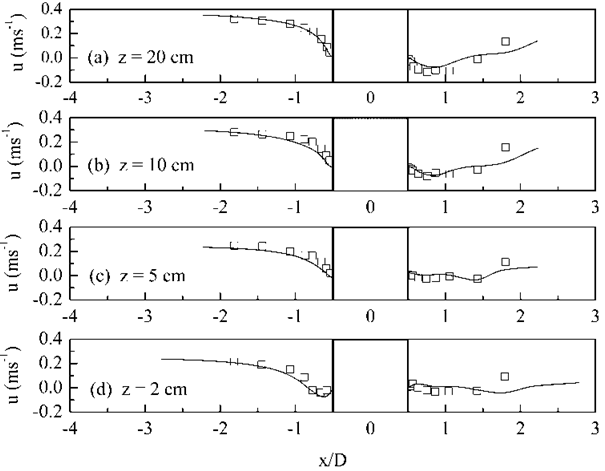Fig. 2. Comparison between the computed and measured horizontal velocity in the plane of symmetry at different distances from the bed.

Fig. 2 shows the computed and measured horizontal velocity in the plane of symmetry at different distances from the bed. Fig. 3 depicts the comparison of the bed shear stress along the x- axis between model results and the experimental data. It should be mentioned that the negative value in Fig. 3 corresponds to the location of the horseshoe vortex in front of the cylinder. It can be found from Figs. 2 and 3 that reasonably good agreement is achieved between the numerical results and the experimental data. Fig. 4 shows the comparison of the amplification of the bed shear stress between the numerical results and the experimental data. Here, the bed shear stress is normalized by the undisturbed bed shear stress. The agreement between the model results.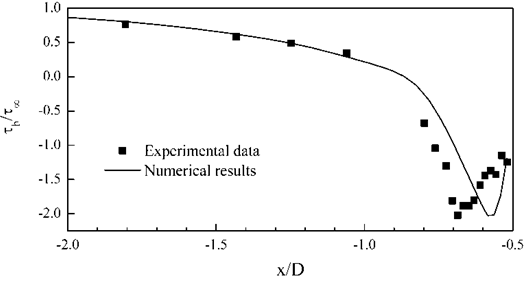Fig. 3. Comparison of the bed shear stress along the x- axis between model results and the experimental data.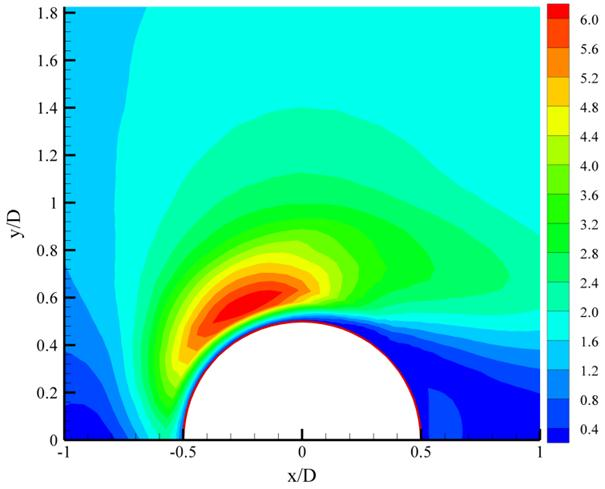Fig. 4. Comparison of the amplification of the bed shear stress between the numerical results and the experimental data.

and the measurements is also reasonable. The maximum value of the bed shear stress is larger than 6.5 and the position of it is near the cylinder surface and located in the radial direction of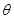= 60°, whereis measured clockwise from the x-axis through the center of the cylinder.

5.2. Bed Shear Stress in Front of a Cylinder Exposed to Waves

In the second test case, to examine the capability of the model to simulate waves interacting with a circular cylinder, the computed bed shear stress was compared with the experimental data obtained by Sumer et al. (2005) . The numerical simulations were conducted in a wave tank with 30 m long and 0.6 m width. A schematic diagram also can be referred to as Fig. 1.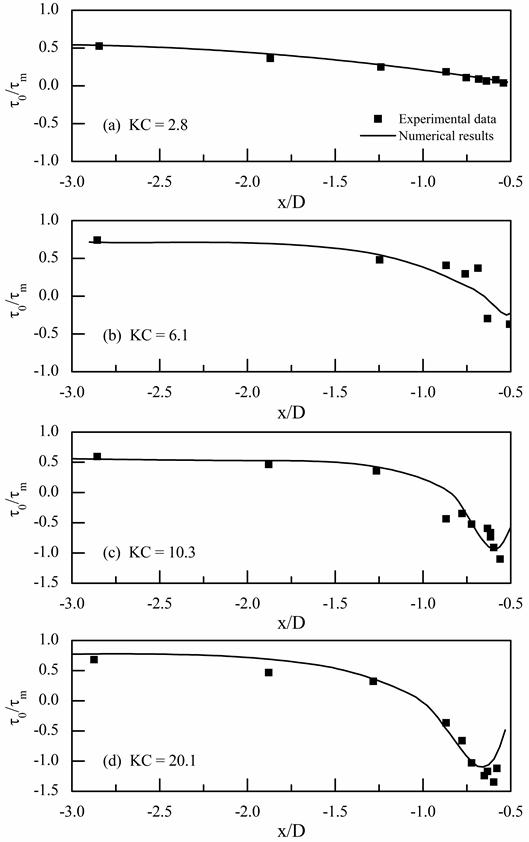Fig. 5. Comparison of the bed shear stress in front of the cylinder between model results and experimental data.

The cylinder diameter was D= 0.04 m. The computational domain is also discretized by horizontal triangular grids plus 40 vertical layers. The horizontal grid spacing is equal to 0.0017 m around the cylinder surface. The incoming regular waves with KC number = 2.8, 6.1, 10.3 and 20.1 were imposed in the upstream boundary, respectively. The corresponding computed bed shear stresses in front of the cylinder were compared with the experimental data, as shown in Fig. 5. Overall, the model reasonably predicts the distribution of the bed shear stress, although it underestimates the maximum value of the bed shear stress for KC number = 6.1, 10.3 and 20.1.

6. Conclusion

In this paper, a numerical model based on 3D Reynolds-averaged Navier-Stokes equations is presented for current or wave interacting with a circular cylinder. An explicit projection method is utilized to solve the governing equations. The computational grid system is built from a two-dimensional horizontal triangular grid by adding a number of horizontal layers. In model validations, generally good agreement between numerical results and experimental data is achieved, demonstrating that the present model with a non-linear k-ε turbulence model is capable of resolving current or wave interacting with a circular cylinder.

Acknowledgements

This work was funded by the National Natural Science Foundation of China (Grant Nos. 51309052, 51479022), the Fundamental Research Funds for the Central Universities (Grant No. DUT15LK01), and the Fundamental Research Project of Key Laboratory of Liaoning Provincial Education Department (Grant No. LZ2015012).

References

1. Ai, C., Jin, S., 2010. Non-hydrostatic finite volume model for non-linear waves interacting with structures. Computers & Fluids 39, 2090-2100.
2. Ai, C., Jin, S., Lv, B., 2011. A new fully non-hydrostatic 3D free surface flow model for water wave motions. International Journal for Numerical methods in Fluids 66, 1354-1370.
3. Ai, C., Jin, S., 2012. A multi-layer non-hydrostatic model for wave breaking and run-up. Coastal Engineering, 62, 1-8.
4. AI, C., Weiye, D., Jin, S., 2014. A general boundary-fitted 3D non-hydrostatic model for nonlinear focusing wave groups. Ocean Engineering, 89, 134-145.
5. Kimura, I., Hosoda, T., 2003. A non-lineark-εmodel with realizability for prediction of flows around bluff bodies. International Journal for Numerical Methods in Fluids 42, 813-837.
6. Li, Y. S., Liu, S.-X., Yu, Y.-X, Lai, G.-Z., 1999. Numerical modeling of Boussinesq equations by finite element method. Coastal Eng. 37, 97-122.
7. Li, Y. S., Zhan, J. M., 2001. Boussinesq-type model with boundary-fitted coordinate system. J. Waterw., Port, Coastal, Ocean Eng. 127, 152-160.
8. Ma, Q. W., Wu, G. X., Eatock Taylor, R., 2001a. Finite element simulation of fully nonlinear interaction between vertical cylinders and steep waves. Part 1: Methodology and numerical procedure. Int. J. Numer. Methods Fluids 36, 265-285.
9. Ma, Q. W., Wu, G. X., Eatock Taylor, R., 2001b. Finite element simulation of fully nonlinear interaction between vertical cylinders and steep waves. Part 2: Numerical results and validation. Int. J. Numer. Methods Fluids 36, 287-308.
10. Ong, M. C., Utnes, T., Holmedal, L. E., Myrhaug, D., Pettersen, B., 2009. Numerical simulation of flow around a smooth circular cylinder at very high Reynolds numbers. Marine Structures 22, 142-153.
11. Roulund A, Sumer B M, Fredsoe J, Michelsen J. Numerical and experimental investigation of flow and scour around a circular pile. Journal of Fluid Mechanics, 2005, 534:351-401.
12. Salih Kirkgoz, M., Alper Oner, A., Sami Akoz, M., 2009. Numerical modeling of interaction of a current with a circular cylinder near a rigid bed. Advances in Engineering Software 40, 1191-1199.
13. Sumer B M, Christiansen N, Fredsoe J. The horseshoe vortex and vortex shedding around a vertical wall-mounted cylinder exposed to waves. Journal of Fluid Mechanics, 1997, 332:41-70.
14. Wang, C. Z., Wu, G. X., 2010. Interactions between fully nonlinear water waves and cylinder arrays in a wave tank. Ocean Eng. 37, 400-417.
15. Zhao, M., Cheng, L., Teng, B., 2007. Numerical simulation of solitary wave scattering by a circular cylinder array. Ocean Eng. 34, 489-499.
16. Zhang, B. J., Zhang, Q. H., Zou, G. L., 2015. 3D numerical simulation of bed shear stress distribution in flow around the circular cylinder. Chinese Journal of Hydrodynamics. 30(3), 306-313.
17. Zhong, Z., Wang, K. H., 2009. Modeling fully nonlinear shallow-water waves and their interactions with cylindrical structures. Comput. Fluids 38, 1018-1025.

 Contents 1. 2. 3. 4. 5. 5.1. 5.2. 6.
Article ToolsAbstractPDF(762K)